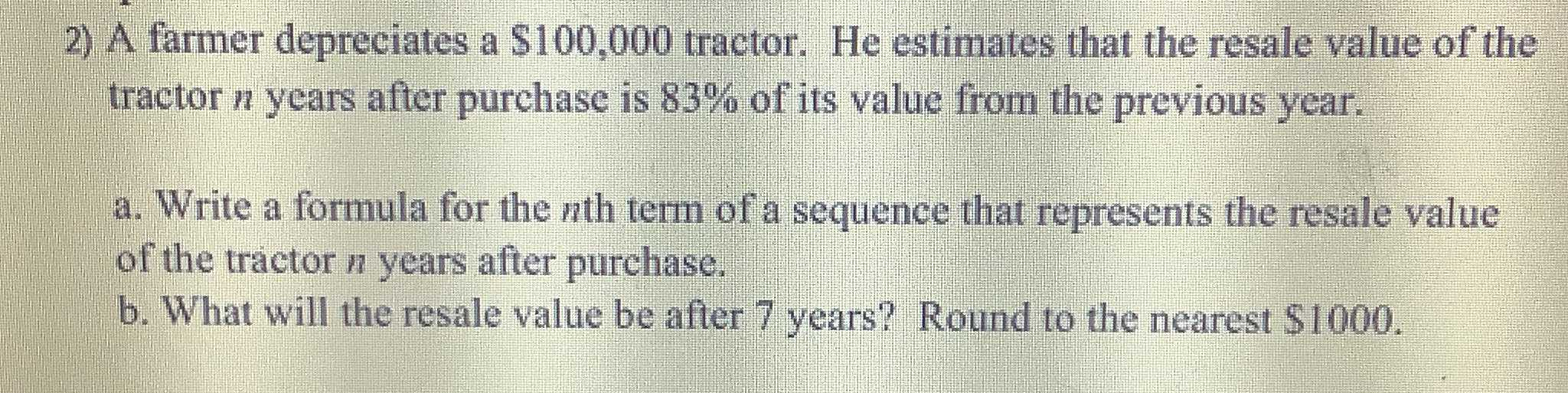### ¿Todavía tienes preguntas de matemáticas?

Pregunte a nuestros tutores expertos
Algebra
Pregunta2) A farmer depreciates a $$\ 100,000$$ tractor. He estimates that the resale value of the tractor $$n$$ years after purchase is $$83 \%$$ of its value from the previous year. a. Write a formula for the $$n$$ th term of a sequence that represents the resale value of the tractor $$n$$ years after purchase. b. What will the resale value be after $$7$$ years? Round to the nearest $$\ 1000$$ .

(a)v=$$100,000\times 0.83^{7}$$